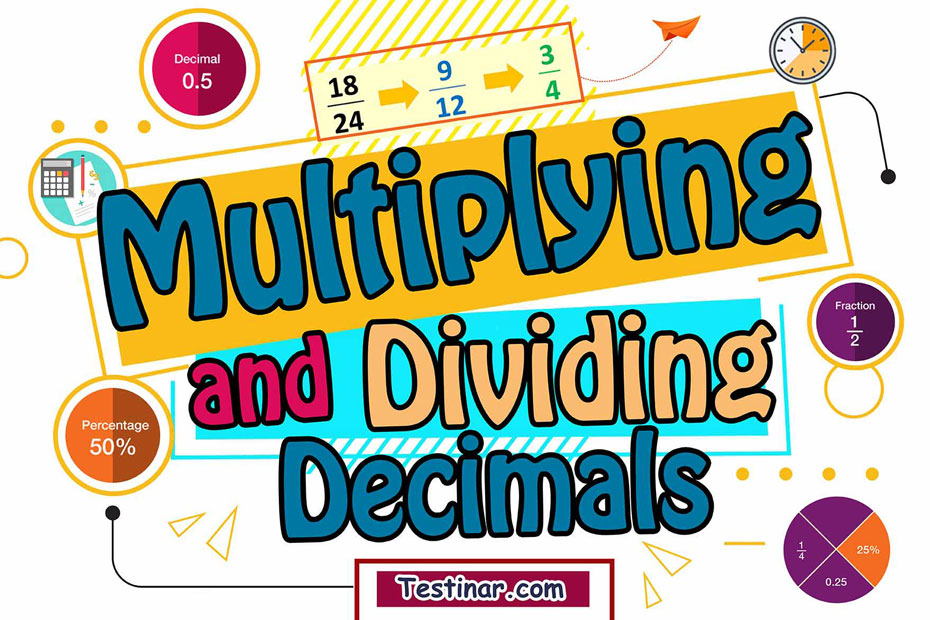## How to Multiply or Divide Decimals

In mathematics, a decimal can be defined as a number which has two parts: a whole part and a fractional part, and these two parts are separated by a decimal point. The whole part always represents a number greater than one, while the fractional part, i.e., the part after the decimal, always represents a number less than one.

For example, let’s take the number $$11.34$$. Now here the whole part is represented by $$11$$, whereas the fractional part $$(\frac{43}{100} \ )$$ is represented by $$43$$. Here $$43$$ can also be denoted as the decimal part, as it lies after the decimal point.

#### Terminating and Non-Terminating Decimals

Terminating decimals are the one which have an end whereas non-terminating decimals don’t have an end. They are simply recurring digits that go on and on.

For example, $$3.333333$$ , $$( \frac{10}{3} \ )$$ is a recurring/non-terminating decimal, whereas $$2.50$$ , $$(\frac{10}{4} \ )$$ is a terminating decimal.

#### The Concept of Preceding Powers of 10

Now, there’s a very interesting concept linked to decimal numbers. This concept is known as the preceding powers of $$10$$. All decimal numbers are based on this concept. So, as we move from left to right in a decimal number, basically, the place value of every digit gets divided by $$10$$. So, the first digit after a decimal could be represented as $$\frac{1}{10}$$ , second as $$\frac{1}{100}$$ and so on.
So, from this concept, we can easily find out the expanded form of a decimal.

For example, let’s take the decimal number $$17.457$$. The expanded form could be written as: $$10 + 7 + \frac{4}{10} + \frac{5}{100} + \frac{7}{1000}$$
Also, $$17.457$$ can be represented as $$17 \frac{457}{1000}$$ in mixed fraction terms.

#### How to Multiply 2 Decimals

To multiply 2 decimals, use the following steps:

• First, line up the two numbers as you would do in case of normal multiplications.
• Now, just forget that they are decimal numbers and proceed with normal multiplication.
• Then add up the decimal points for both numbers. For example, in case of $$2.89 \times 3.74$$, the addition should be $$4$$.
• Now, after normal multiplication, place the decimal point. So, if addition of decimal points is $$4$$, add decimal after 4 digits starting from the extreme right to left.

#### How to Divide 2 Decimals

To divide 2 decimals, use the following steps:

• First, convert the divisor as well as the dividend into a whole number.
• Then divide as normal between two whole numbers.

For example, consider $$(34.75 \div 12.6)$$. Now, multiply both the divisor and dividend by $$100$$ as the largest decimal point is that of hundredth. Now divide $$(3475 \div 1260)$$ as you would normally do.

### Exercises for Multiplying and Dividing Decimals

1) $$12.8\ \div \ 5.7 \ =$$

2) \cfrac{\times\begin{align}27.8\\17.3\end{align}}{}

3) $$88.8\ \div \ 41.6 \ = \$$

4) \cfrac{\times\begin{align}76.6\\24.2\end{align}}{}

5) $$49.4\ \div \ 21.2 \ = \$$

6) $$27.8\ \div \ 1.2 \ = \$$

7) $$43.6\ \div \ 34.2 \ = \$$

8) \cfrac{\times\begin{align}61.6\\39.5\end{align}}{}

9) \cfrac{\times\begin{align}46.2\\22.5\end{align}}{}

10) $$29.6\ \div \ 17.3 \ =$$

1) $$12.8\ \div \ 5.7 \ = \ \color{red}{2.2456...}$$
Solution
The divisor is not a whole number. Therefore, multiply it by $$10$$ to get $$128$$. Do the same for the dividend to get $$57$$ .
Now, divide: $$128\ \div \ 57 \ = \ 2.2456...$$  . so the answer is $$2.2456...$$  .
2) \cfrac{\times\begin{align}27.8\\17.3\end{align}}{\color{red}{\begin{align}8.34\\194.60\\278.00\end{align} \over 480.94}}
Solution
Set up and multiply the numbers as you do with whole numbers. $$278 \ \times 173 \ = \ 48094$$ . Count the total number of decimal places in both of the factors $$1+1 =2$$ . Then Place the decimal point in the product: $$480.94$$
3) $$88.8\ \div \ 41.6 \ = \ \color{red}{2.1346...}$$
Solution
The divisor is not a whole number. Therefore, multiply it by $$10$$ to get $$888$$. Do the same for the dividend to get $$416$$ .
Now, divide: $$888\ \div \ 416 \ = \ 2.1346...$$ . so the answer is $$2.1346...$$  .
4) \cfrac{\times\begin{align}76.6\\24.2\end{align}}{\color{red}{\begin{align}15.32\\306.40\\1532.00\end{align} \over 1853.72}}
Solution
Set up and multiply the numbers as you do with whole numbers. $$766 \ \times 242 \ = \ 185372$$ . Count the total number of decimal places in both of the factors $$1+1 =2$$ . Then Place the decimal point in the product: $$1853.72$$
5) $$49.4\ \div \ 21.2 \ = \ \color{red}{2.3302...}$$

6) $$27.8\ \div \ 1.2 \ = \ \color{red}{23.1667...}$$

7) $$43.6\ \div \ 34.2 \ = \ \color{red}{1.2749...}$$

8) \cfrac{\times\begin{align}61.6\\39.5\end{align}}{\color{red}{\begin{align}30.80\\554.40\\1848.00\end{align} \over 2433.2}}

9) \cfrac{\times\begin{align}46.2\\22.5\end{align}}{\color{red}{\begin{align}23.10\\92.40\\924.00\end{align} \over 1039.5}}

10) $$29.6\ \div \ 17.3 \ = \ \color{red}{1.711...}$$

## Multiply and Divide Decimals Quiz

### Prepare for the AFOQT Math Test in 7 Days

$25.99$14.99

### TSI Math for Beginners 2022

$24.99$14.99

### Praxis Math Formulas

$6.99$5.99

### HSPT Math for Beginners

$24.99$14.99